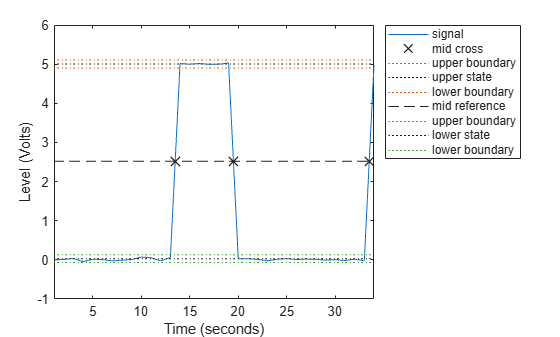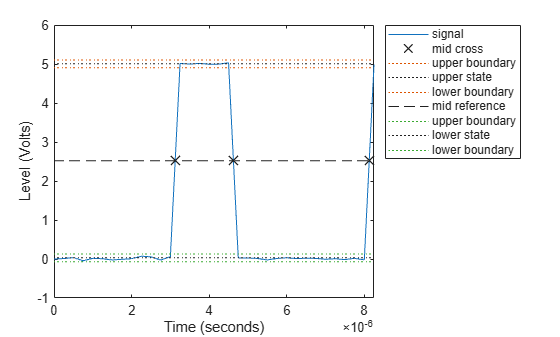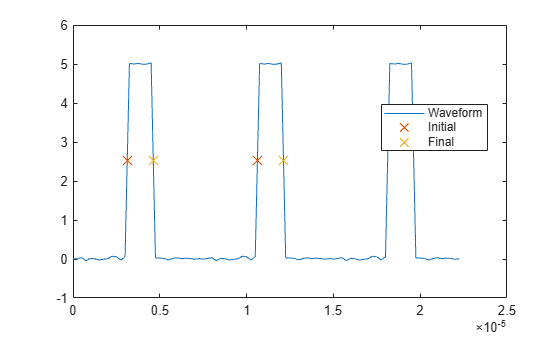Documentation

dutycycle

Duty cycle of pulse waveform

Syntax

D = dutycycle(X)
D = dutycycle(X,FS)
D = dutycycle(X,T)
D = dutycycle(TAU,PRF)
[D,INITCROSS] = dutycycle(X,...)
[D,INITCROSS,FINALCROSS] = dutycycle(X,...)
[D,INITCROSS,FINALCROSS,NEXTCROSS] = dutycycle(X,...)
[D,INITCROSS,FINALCROSS,NEXTCROSS,MIDLEV] = dutycycle(X,...)
[D,INITCROSS,FINALCROSS,NEXTCROSS] = dutycycle(X,...,Name,Value)
dutycycle(X,...)

Description

D = dutycycle(X) returns the ratio of pulse width to pulse period for each positive-polarity pulse. D has length equal to the number of pulse periods in X. The sample instants of X correspond to the indices of X. To determine the transitions that define each pulse, dutycycle estimates the state levels of the input waveform by a histogram method. dutycycle identifies all regions, which cross the upper-state boundary of the low state and the lower-state boundary of the high state. The low-state and high-state boundaries are expressed as the state level plus or minus a multiple of the difference between the state levels. See State-Level Tolerances.

D = dutycycle(X,FS) specifies the sample rate, FS, in hertz as a positive scalar. The first sample instant of X corresponds to t=0.

D = dutycycle(X,T) specifies the sample instants, T, as a vector with the same number of elements as X.

D = dutycycle(TAU,PRF) returns the ratio of pulse width to pulse period for a pulse width of TAU seconds and a pulse repetition frequency of PRF. The product of TAU and PRF must be less than or equal to 1.

[D,INITCROSS] = dutycycle(X,...) returns a vector, INITCROSS, whose elements correspond to the mid-crossings (mid-reference level instants) of the initial transition of each pulse with a corresponding NEXTCROSS.

[D,INITCROSS,FINALCROSS] = dutycycle(X,...) returns a vector, FINALCROSS, whose elements correspond to the mid-crossings (mid-reference level instants) of the final transition of each pulse with a corresponding NEXTCROSS.

[D,INITCROSS,FINALCROSS,NEXTCROSS] = dutycycle(X,...) returns a vector, NEXTCROSS, whose elements correspond to the mid-crossings (mid-reference level instants) of the next detected transition for each pulse.

[D,INITCROSS,FINALCROSS,NEXTCROSS,MIDLEV] = dutycycle(X,...) returns the mid-reference level, MIDLEV. Because in a bilevel pulse waveform the state levels are constant, MIDLEV is a scalar.

[D,INITCROSS,FINALCROSS,NEXTCROSS] = dutycycle(X,...,Name,Value) returns the ratio of pulse width to pulse period with additional options specified by one or more Name,Value pair arguments.

dutycycle(X,...) plots the waveform, X, and marks the location of the mid-reference level instants and the associated reference levels. The state levels and associated lower and upper state boundaries are also plotted.

Input Arguments

 X Bilevel waveform. X is a real-valued row or column vector. FS Sample rate in hertz. T Vector of sample instants. The length of T must equal the length of the bilevel waveform, X. TAU Pulse width in seconds. The product of TAU and PRF must be less than or equal to 1. PRF Pulse repetition frequency in pulses/second. The product of TAU and PRF must be less than or equal to 1.

Name-Value Pair Arguments

 'MidPercentReferenceLevel' Mid-reference level as a percentage of the waveform amplitude. Default: 50 'Polarity' Pulse polarity. Specify the polarity as 'positive' or 'negative'. If you specify 'positive', dutycycle looks for pulses with positive-going (positive polarity) initial transitions. If you specify 'negative', dutycycle looks for pulses with negative-going (negative polarity) initial transitions. See Pulse Polarity for examples of positive and negative-polarity pulses. Default: 'positive' 'StateLevels' Low- and high-state levels. StateLevels is a 1-by-2 real-valued vector. The first element is the low-state level. The second element is the high-state level. If you do not specify low- and high-state levels, dutycycle estimates the state levels from the input waveform using the histogram method. 'Tolerance' Tolerance levels (lower- and upper-state boundaries) expressed as a percentage. See State-Level Tolerances. Default: 2

Output Arguments

 D Duty cycle. Duty cycle is the ratio of the pulse width to the pulse period. Because the pulse width cannot exceed the pulse period, 0≤D ≤1. INITCROSS Mid-reference level instant of initial transition. Because the duty cycle is defined as the ratio of pulse width to pulse period, initial transitions are only reported when dutycycle finds a corresponding NEXTCROSS. FINALCROSS Mid-reference level instant of final transition. The duty cycle is defined as the ratio of pulse width to pulse period. Thus, final transitions are only reported when dutycycle finds a corresponding NEXTCROSS. NEXTCROSS Mid-reference level instant of the first initial transition after the final transition of the preceding pulse. MIDLEV Mid-reference level. The waveform value that corresponds to the mid-reference level.

Examples

collapse all

Determine the duty cycle of a bilevel waveform. Use the vector indices as the sample instants.

d = dutycycle(x)
d = 0.3001

Annotate the result on a plot of the waveform.

dutycycle(x);Determine the duty cycle of a bilevel waveform. The sample rate is 4 MHz.

fs = 1/(t(2)-t(1));

d = dutycycle(x,fs)
d = 0.3001

Annotate the result on a plot of the waveform.

dutycycle(x,fs);Create a pulse waveform with three pulses. The sample rate is 4 MHz. Determine the initial and final mid-reference level instants. Plot the result.

fs = 4e6;

pulse = x(1:30);
wavef = [pulse;pulse;pulse];
t = (0:length(wavef)-1)/fs;

[~,initcross,finalcross,~,midlev] = dutycycle(wavef,t)
initcross = 2×1
10-4 ×

0.0312
0.1062

finalcross = 2×1
10-4 ×

0.0463
0.1213

midlev = 2.5177

Even though there are three pulses, only two pulses have corresponding subsequent transitions. Plot the result.

plot(t,wavef)
hold on
plot([initcross finalcross],midlev*ones(2),'x','markersize',10)
hold off
legend('Waveform','Initial','Final','Location','best')collapse all

Duty Cycle

The energy in a bilevel, or rectangular, pulse is equal to the product of the peak power, Pt, and the pulse width, τ. Devices to measure energy in a waveform operate on time scales longer than the duration of a single pulse. Therefore, it is common to measure the average power

${P}_{\text{av}}=\frac{{P}_{t}\tau }{T},$

where T is the pulse period.

The ratio of average power to peak power is the duty cycle:

$D=\frac{{P}_{t}\tau /T}{{P}_{t}}$

Pulse Polarity

If the pulse has a positive-going initial transition, the pulse has positive polarity. The following figure shows a positive polarity pulse.Equivalently, a positive-polarity (positive-going) pulse has a terminating state more positive than the originating state.

If the pulse has a negative-going initial transition, the pulse has negative polarity. The following figure shows a negative-polarity pulse.Equivalently, a negative-polarity (negative-going) pulse has a originating state more positive than the terminating state.

State-Level Tolerances

Each state level can have associated lower- and upper-state boundaries. These state boundaries are defined as the state level plus or minus a scalar multiple of the difference between the high state and the low state. To provide a useful tolerance region, the scalar is typically a small number such as 2/100 or 3/100. In general, theregion for the low state is defined aswhereis the low-state level andis the high-state level. Replace the first term in the equation withto obtain thetolerance region for the high state.

The following figure illustrates lower and upper 2% state boundaries (tolerance regions) for a positive-polarity bilevel waveform. The red dashed lines indicate the estimated state levels.References

 Skolnik, M. I. Introduction to Radar Systems. New York, NY: McGraw-Hill, 1980.

 IEEE® Standard on Transitions, Pulses, and Related Waveforms. IEEE Standard 181, 2003.